# Largest Element in the BST less than or Equal to N

In this article, we are going to see how to find the largest element in the BST which is less than or equal to N?
Submitted by Radib Kar, on November 02, 2020

In this article, we are going to see how to find the largest element in a given Binary Search Tree which is less than or equal to a given input number, N.

For example,

In the below tree,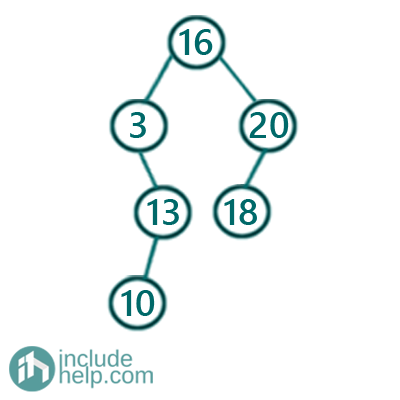If given number, N is 15, then the largest in the binary search tree is 13 which is less than or equal to 15.

If given number, N is 1, then there is no number which is either equal or less than 1.

So, to solve this basically, we have to keep searching. The idea is like below:

1. Initially result with INT_MIN which will store the maximum possible element maintaining the constraint.
3. If current node value is equal to N, then we have got our maximum possible value which is N itself.
4. If current node value is less than N than the maximum possible value under the constraint till now is the current node value. So update result with current node value and move towards the right subtree to search if there is a larger number satisfying the constraints.
5. If current node value is more than N, then we can't update the result, as we are not sure yet if there exists any value less than or equal to N. So we will move towards left subtree to find if there is any element or not.

Below is the C++ implementation:

```#include <bits/stdc++.h>
using namespace std;

// tree node is defined
class TreeNode {
public:
int val;
TreeNode* left;
TreeNode* right;
TreeNode(int data)
{
val = data;
left = NULL;
right = NULL;
}
};

void searchLargest(TreeNode* root, int& result, int n)
{
if (!root)
return;

if (root->val == n) {
//so since n exists itself in the binary tree
result = root->val;
return;
}
//since current node is less than n we can move right
else if (root->val < n) {
//but for now, maximum valus less than n is current node value
//so update that
result = root->val;
searchLargest(root->right, result, n);
}
//current node value is more than n, so move to left,
//till now maxium is same as what we have already(INT_MIN)
else
return searchLargest(root->left, result, n);
}

int LargestNumberlessThanEqualToN(TreeNode* root, int n)
{
int result = INT_MIN;

searchLargest(root, result, n);

return result;
}

int main()
{
TreeNode* root = new TreeNode(16);
root->left = new TreeNode(3);
root->left->right = new TreeNode(13);
root->left->right->left = new TreeNode(10);
root->right = new TreeNode(20);
root->right->left = new TreeNode(18);
int n = 15;
int maximum = LargestNumberlessThanEqualToN(root, n);
if (maximum != INT_MIN)
cout << "Largest value in the BST less than 15 is: " << maximum << endl;
else
cout << "There is no largest value less than or equal to N\n";
n = 1;
maximum = LargestNumberlessThanEqualToN(root, n);
if (maximum != INT_MIN)
cout << "Largest value in the BST less than 15 is: " << maximum << endl;
else
cout << "There is no largest value less than or equal to N\n";

return 0;
}
```

Output:

```Largest value in the BST less than 15 is: 13
There is no largest value less than or equal to N
```

Dry Run:

Below is the dry run for 1st example where N is 15.

1. So firstly, we start with the root. The root is greater than N(15), so we need to move towards the left subtree and we can't update result now. Thus result is still INT_MIN.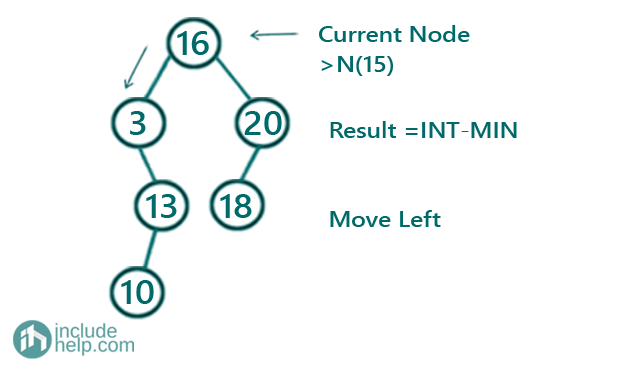2. Now, the current node is 3, thus we update result as 3 and try to find if there is any larger element possible. Thus we move towards right subtree. So, result is now 3.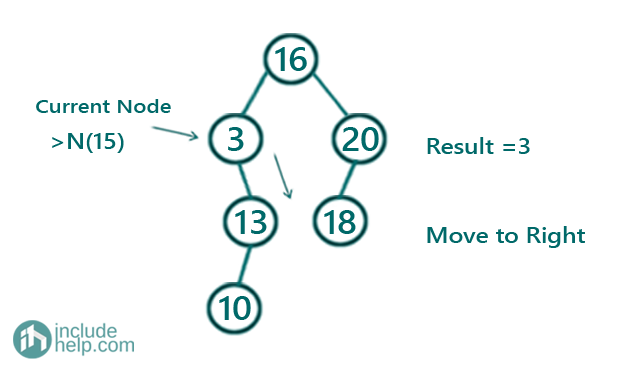3. Now, the current node is 13 which is still less than 15, thus we update result as 13 and try to find if there is any larger element possible. Thus we move towards right subtree. So, result is now 13.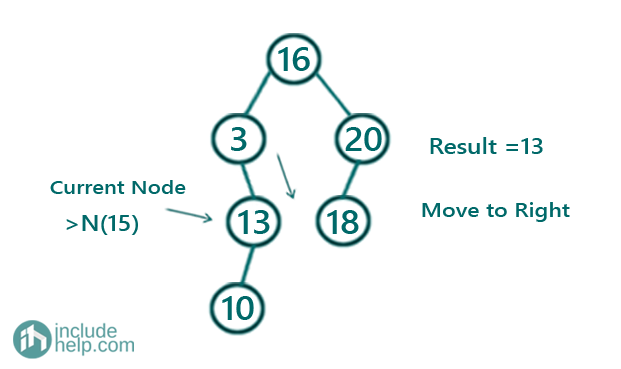4. Now root is NULL, so our final answer is 13.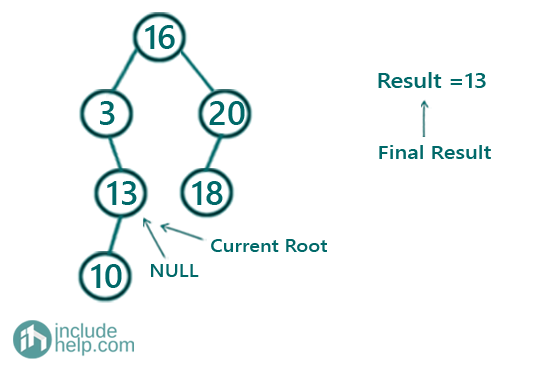For the second example, where N is 1, you can do the dry run and we will find we don't find any node which is less than or equal to 1.

Top MCQs